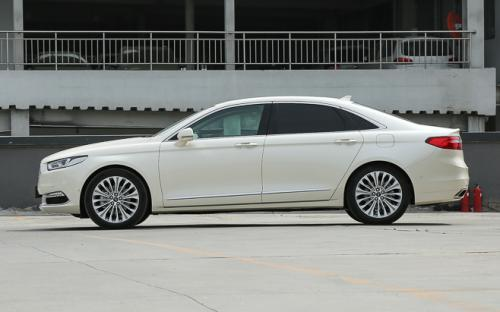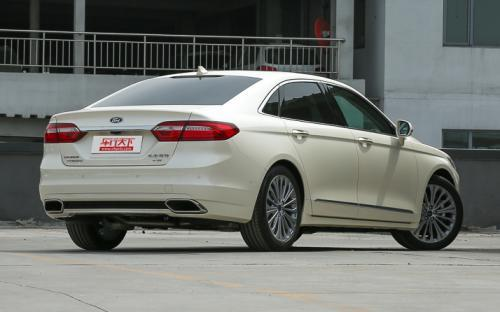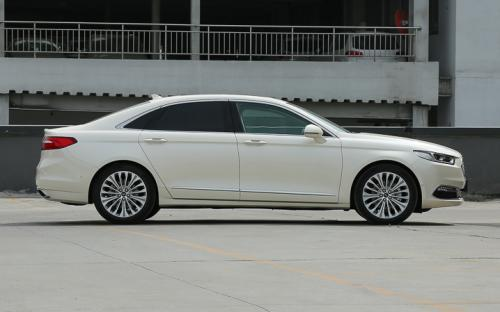### 长安福特 金牛座0 种颜色可选2017款最低售价：23.48 万元起

4996(mm)1878(mm)1503(mm)##### 配置亮点：
• 胎压监测装置

• ISOFIX儿童座椅接口

• 车身稳定控制(ESC/ESP/DSC等)

• 电动天窗

• 定速巡航

• 后倒车雷达

• 真皮座椅

• GPS导航系统

• 氙气大灯

• 后视镜加热

• 提交
2017款 EcoBoost 180 豪华型 (83张)
• 2017款 EcoBoost 180 豪华型 (83张)
• 2017款 EcoBoost 325 V6 LTD限量版 (347张)
• 长安福特 金牛座 绕车实拍• 长安福特 金牛座 在售车型

排量 车型 厂商指导价 本地最低报价 购车工具
1.5T
EcoBoost 180 豪华型 6挡手自一体
23.48万
EcoBoost 245 时尚型 6挡手自一体
24.98万
EcoBoost 245 豪华型 6挡手自一体
26.98万
EcoBoost 245 旗舰型 6挡手自一体
29.98万
EcoBoost 325 V6旗舰型 6挡手自一体
34.98万
EcoBoost 325 V6 LTD限量版 6挡手自一体
36.98万
2.0T
EcoBoost 180 豪华型 6挡手自一体
23.48万
EcoBoost 245 时尚型 6挡手自一体
24.98万
EcoBoost 245 豪华型 6挡手自一体
26.98万
EcoBoost 245 旗舰型 6挡手自一体
29.98万
EcoBoost 325 V6旗舰型 6挡手自一体
34.98万
EcoBoost 325 V6 LTD限量版 6挡手自一体
36.98万
2.7T
EcoBoost 180 豪华型 6挡手自一体
23.48万
EcoBoost 245 时尚型 6挡手自一体
24.98万
EcoBoost 245 豪华型 6挡手自一体
26.98万
EcoBoost 245 旗舰型 6挡手自一体
29.98万
EcoBoost 325 V6旗舰型 6挡手自一体
34.98万
EcoBoost 325 V6 LTD限量版 6挡手自一体
36.98万

长安福特 金牛座 经销商

查看更多 >>

### 长安福特 金牛座 动力加速

金牛座 0-100公里加速时间分布在 6.9-10.6秒 属于 高性能级

动力级别 加速时间 车型
民用级(1款)
10.6s
EcoBoost 180 豪华型
运动级(1款)
7.9s
EcoBoost 245 时尚型EcoBoost 245 豪华型EcoBoost 245 旗舰型
高性能级(1款)
6.9s
EcoBoost 325 V6旗舰型EcoBoost 325 V6 LTD限量版

长安福特 金牛座 视频

长安福特 金牛座 新闻资讯

# 福特2017款金牛座上市 售价23.48万起

上市新车 超过8168次关注

近日，长安福特官方宣布旗下2017款金牛座车型正式上市。新车分为3种排量共计6款车型，售价区间为23.48-36.98万元。新车在车型和配置方面进行了改进。

# 闷骚的VIP 测试金牛座2.0L GTDi智尊型

试驾评测 超过9449次关注

长安福特在迈向中大型车市场的首款力作，24.88-34.88万元的售价区间也使其在上市之后就和别克君越、丰田皇冠等车型形成了竞争的势态；今天我们要给大家展示的2.0T车型。

# 2015广州车展 福特金牛座正式上市

上市新车 超过9164次关注

本届广州车展上，长安福特宣布金牛座正式上市，运用福特家族式六边形多辐格栅设计，新车将提供两种动力共计五款车型选择，前保险杠两侧格栅融入了LED日间行车灯，提...

# 9月上市 长安福特金牛座预售24.98万起

即将上市 超过7180次关注

长安福特官方发布了国产金牛座车型的预售价格，新车从即日起启动预售。定位于中大型车，采用了福特家族最新的设计风格；将搭载2.0T和2.7T两种发动机，将推出5款车型...

# 9月底上市 长安福特金牛座正式下线

国产新车 超过7168次关注

长安福特金牛座正式下线，定位为一款中大型车，新车在外观上运用了福特家族最新的六边形前进气格栅，整体造型庄重大气。内部延续了较为稳重的设计风格，搭载了1.5T...

# 金牛座等 福特下半年将在国内推5款新车

行业动态 超过8319次关注

福特官方称，将在2015下半年投放5款新车型，包括福特金牛座、撼路者、全新探险者、新款福克斯和新款福克斯ST，助力福特在华2015下半年销量增长随着下半年为中国市场...

猜你喜欢

﻿
• 快速找车
• 选择品牌
• 选择品牌
• A  奥迪
• A  阿斯顿·马丁
• A  阿尔法·罗密欧
• B  宝沃
• B  布加迪
• B  巴博斯
• B  保时捷
• B  宾利
• B  奔驰
• B  宝马
• B  本田
• B  别克
• B  标致
• B  比亚迪
• B  宝骏
• B  北汽制造
• B  北汽新能源
• B  北汽幻速
• B  北汽威旺
• B  北京汽车
• B  奔腾
• B  北汽绅宝
• C  长安
• C  长安商用
• C  长城
• C  昌河
• D  大众
• D  道奇
• D  DS
• D  东南
• D  东风风神
• D  东风风行
• D  东风小康
• D  东风风度
• D  东风
• F  福特
• F  丰田
• F  菲亚特
• F  法拉利
• F  福田
• F  福迪
• F  福汽启腾
• G  观致
• G  广汽传祺
• G  广汽吉奥
• G  GMC
• H  红旗
• H  汉腾汽车
• H  哈弗
• H  哈飞
• H  海格
• H  海马
• H  华颂
• H  黄海
• H  华泰
• H  恒天
• J  吉利汽车
• J  捷豹
• J  Jeep
• J  江淮
• J  江铃
• J  金杯
• J  九龙
• J  金旅
• K  凯翼
• K  凯迪拉克
• K  克莱斯勒
• K  科尼塞克
• K  卡威
• K  开瑞
• L  路虎
• L  林肯
• L  劳斯莱斯
• L  兰博基尼
• L  雷克萨斯
• L  铃木
• L  雷诺
• L  理念
• L  力帆
• L  莲花汽车
• L  猎豹
• L  路特斯
• L  陆风
• M  马自达
• M  MG
• M  MINI
• M  玛莎拉蒂
• M  摩根
• M  迈凯轮
• N  纳智捷
• O  欧宝
• O  讴歌
• O  欧朗
• Q  奇瑞
• Q  起亚
• Q  启辰
• R  日产
• R  荣威
• R  瑞麒
• S  三菱
• S  斯威汽车
• S  萨博
• S  smart
• S  斯柯达
• S  斯巴鲁
• S  思铭
• S  双龙
• S  上汽大通
• S  双环
• T  特斯拉
• T  腾势
• W  沃尔沃
• W  五菱汽车
• W  五十铃
• W  威兹曼
• W  威麟
• X  现代
• X  雪佛兰
• X  雪铁龙
• X  西雅特
• Y  一汽
• Y  英菲尼迪
• Y  英致
• Y  依维柯
• Y  野马汽车
• Y  永源
• Z  众泰
• Z  中华
• Z  中兴
• Z  知豆
• 选择车系
• 选择车系
• 车型对比
• 选择品牌
• 选择品牌
• A  奥迪
• A  阿斯顿·马丁
• A  阿尔法·罗密欧
• B  宝沃
• B  布加迪
• B  巴博斯
• B  保时捷
• B  宾利
• B  奔驰
• B  宝马
• B  本田
• B  别克
• B  标致
• B  比亚迪
• B  宝骏
• B  北汽制造
• B  北汽新能源
• B  北汽幻速
• B  北汽威旺
• B  北京汽车
• B  奔腾
• B  北汽绅宝
• C  长安
• C  长安商用
• C  长城
• C  昌河
• D  大众
• D  道奇
• D  DS
• D  东南
• D  东风风神
• D  东风风行
• D  东风小康
• D  东风风度
• D  东风
• F  福特
• F  丰田
• F  菲亚特
• F  法拉利
• F  福田
• F  福迪
• F  福汽启腾
• G  观致
• G  广汽传祺
• G  广汽吉奥
• G  GMC
• H  红旗
• H  汉腾汽车
• H  哈弗
• H  哈飞
• H  海格
• H  海马
• H  华颂
• H  黄海
• H  华泰
• H  恒天
• J  吉利汽车
• J  捷豹
• J  Jeep
• J  江淮
• J  江铃
• J  金杯
• J  九龙
• J  金旅
• K  凯翼
• K  凯迪拉克
• K  克莱斯勒
• K  科尼塞克
• K  卡威
• K  开瑞
• L  路虎
• L  林肯
• L  劳斯莱斯
• L  兰博基尼
• L  雷克萨斯
• L  铃木
• L  雷诺
• L  理念
• L  力帆
• L  莲花汽车
• L  猎豹
• L  路特斯
• L  陆风
• M  马自达
• M  MG
• M  MINI
• M  玛莎拉蒂
• M  摩根
• M  迈凯轮
• N  纳智捷
• O  欧宝
• O  讴歌
• O  欧朗
• Q  奇瑞
• Q  起亚
• Q  启辰
• R  日产
• R  荣威
• R  瑞麒
• S  三菱
• S  斯威汽车
• S  萨博
• S  smart
• S  斯柯达
• S  斯巴鲁
• S  思铭
• S  双龙
• S  上汽大通
• S  双环
• T  特斯拉
• T  腾势
• W  沃尔沃
• W  五菱汽车
• W  五十铃
• W  威兹曼
• W  威麟
• X  现代
• X  雪佛兰
• X  雪铁龙
• X  西雅特
• Y  一汽
• Y  英菲尼迪
• Y  英致
• Y  依维柯
• Y  野马汽车
• Y  永源
• Z  众泰
• Z  中华
• Z  中兴
• Z  知豆
• 选择车系
• 选择车系
• 选择车型
• 选择车型
• 意见反馈